×

Web Demos

Explore the flexibe barcode reading settings to optimize for your specific usage scenario.

WEB TWAIN SDK DEMO

Try the most popular web scanner features: scan images, import local images and PDF files, edit, save to local, upload to database, and etc.

Transform any camera-equipped devices into real-time, browser-based barcode and QR code scanners.

MRZ SCANNER WEB DEMO

Detects the machine-readable zone of a passport, scans the text, and parses into human-readable data.

APP STORE DEMOS

VIEW MORE DEMOS >

# Image Processing 101 Chapter 2.2: Point Operations

Jun 07, 2019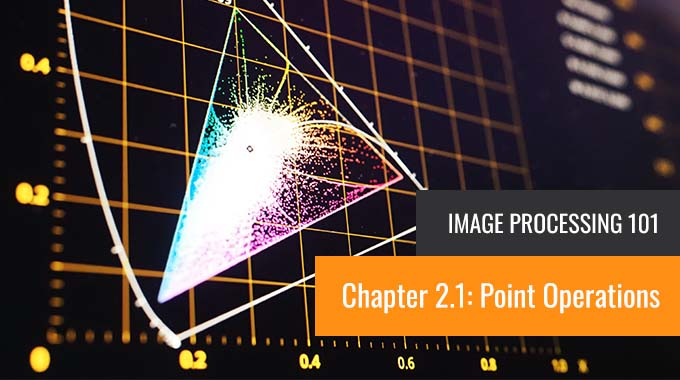This post discusses the basic point operations and the next post will be covering spatial filters.

## Point Operation

Point operations are often used to change the grayscale range and distribution. The concept of point operation is to map every pixel onto a new image with a predefined transformation function.

``````g(x, y) = T(f(x, y))
``````
• g (x, y) is the output image
• T is an operator of intensity transformation
• f (x, y) is the input image

## Basic Intensity Transformation Functions

The simplest image enhancement method is to use a 1 x 1 neighborhood size. It is a point operation. In this case, the output pixel (‘s’) only depends on the input pixel (‘r’), and the point operation function can be simplified as follows:

``````s = T(r)
``````

Where T is the point operator of a certain gray-level mapping relationship between the original image and the output image.

• s,r: denote the gray level of the input pixel and the output pixel.

Different transformation functions work for different scenarios.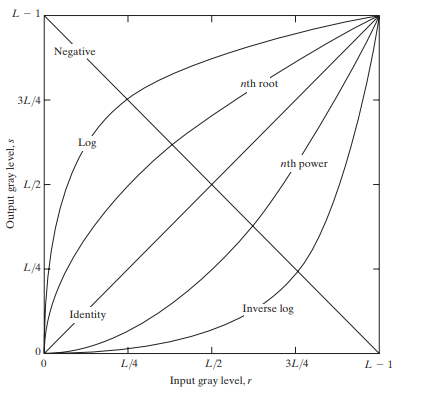Image source: Slideshare.net

### Linear

Linear transformations include identity transformation and negative transformation.

In identity transformation, the input image is the same as the output image.

``````s = r
``````

The negative transformation is:

``````s = L - 1 - r = 256 - 1 - r = 255 - r
``````

L = Largest gray level in an image. The negative transformation is suited for enhancing white or gray detail embedded in dark areas of an image, for example, analyzing the breast tissue in a digital mammogram.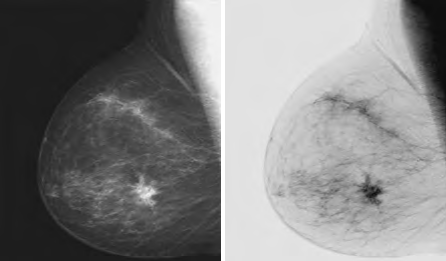Image source: Slideshare.net

### Logarithmic

a) General Log Transform

The equation of general log transformation is:

``````s = c * log(1 + r)
``````

Note:

• s,r: denote the gray level of the input pixel and the output pixel.
• ‘c’ is a constant; to map from [0,255] to [0,255], c = 255/LOG(256)
• the base of a common logarithm is 10

In the log transformation, the low-intensity values are mapped into higher intensity values. It maps a narrow range of low gray levels to a much wider range. Generally speaking, the log transformation works the best for dark images.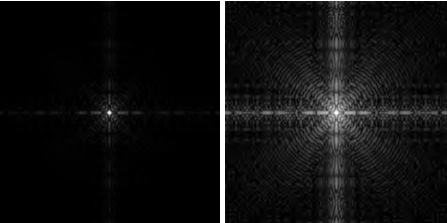Image source: Slideshare.net

b) Inverse Log Transform

The inverse log transform is opposite to log transform. It maps a narrow range of high gray levels to a much wider range. The inverse log transform expands the values of light-level pixels while compressing the darker-level values.

``````s = power(10, r * c)-1
``````

Note:

• s,r: denote the gray level of the input pixel and the output pixel.
• ‘c’ is a constant; to map from [0,255] to [0,255], c =LOG(256)/255

### Power-Law (Gamma) Transformation

The gamma transform, also known as exponential transformation or power transformation, is a commonly used grayscale non-linear transformation. The mathematical expression of the gamma transformation is as follows:

s = c * power(r, γ), where

• s,r: denote the gray level of the input pixel and the output pixel;
• ‘c’ is a constant
• ‘γ’ is also a constant and is the gamma coefficient.

Plots of the equation [formula] for various values of γ (c = 1 in all cases)

All curves were scaled to fit in the range shown. X-axis represents input intensity level ‘r’, and y-axis represents the output intensity level ‘s’.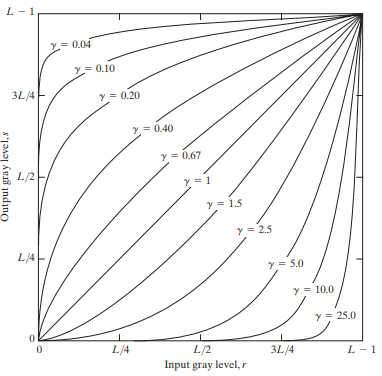Image source: Slideshare.net

When performing the conversion, it is a common practice to first convert the intensity level from the range of 0 to 255 to 0 to 1. Then perform the gamma conversion and at last restore to the original range.

Comparing to log transformation, gamma transformation can generate a family of possible transformation curves by varying the gamma value.

Here are the enhanced images output by using different γ (gamma) values.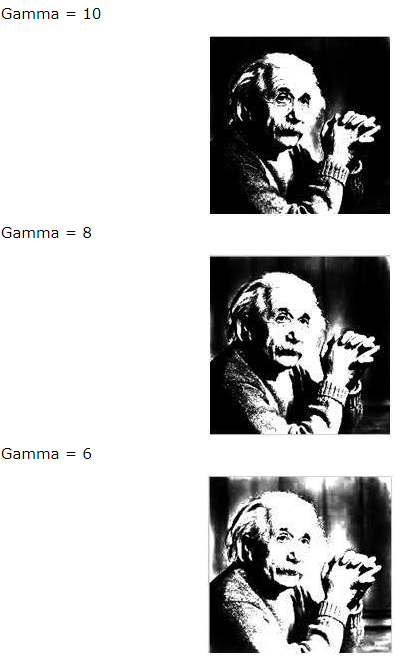Image source: TutorialsPoint.com

The gamma transformation can selectively enhance the contrast of the dark region or the light region depending on the value of γ.

• When γ > 1, the contrast of the light gray area is enhanced. Take γ = 25 for example, the pixels with the range of 0.8-1 (at the scale of 256, it corresponds to 240-255) are mapped to the range of 0-1
• When γ < 1, the contrast of the dark gray area is enhanced
• When γ = 1, this transformation is linear, that is, the original image is not changed

## Piecewise Transformation

In mathematics, a piecewise-defined function is a function defined by multiple sub-functions, where each sub-function applies to a different interval in the domain.

Types of piecewise transformations:

• Grayscale Threshold Transform (Gray-level Slicing)
• Contrast Stretching
• Bit-plane Slicing

### Grayscale Threshold Transform

Grayscale Threshold Transform converts a grayscale image into a black and white binary image. The user specifies a value that acts as a dividing line. If the gray value of a pixel is smaller than the dividing, the intensity of the pixel is set to 0, otherwise it’s set to 255. The value of the dividing line is called the threshold. The grayscale threshold transform is often referred to as thresholding, or binarization.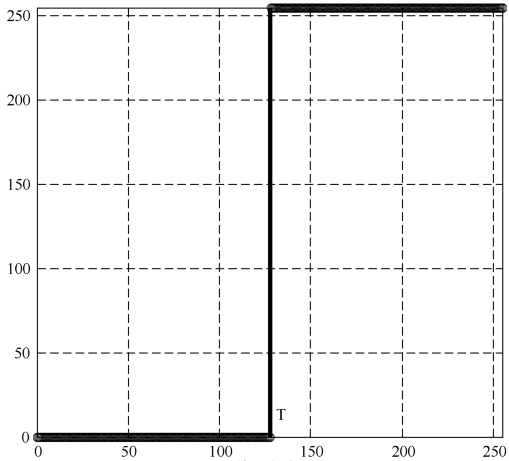### Contrast-Stretching Transformation

The goal of the contrast-stretching transformation is to enhance the contrast between different parts of an image, that is, enhances the gray contrast for areas of interest, and suppresses the gray contrast for areas that are not of interest.

Below are two possible functions:

1. Power-Law Contrast-Stretching Transformation

If T(r) has the form as shown in the figure, the effect of applying the transformation to every pixel to generate the corresponding pixels produce higher contrast than the original image, by:

• Darkening the levels below k in the original image
• Brightening the levels above k in the original image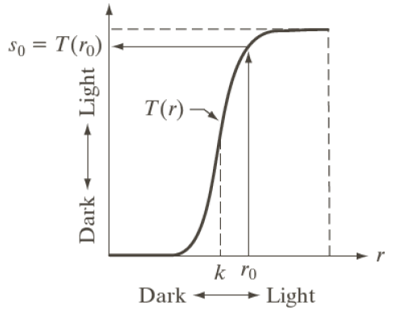2. Linear Contrast-Stretching Transformation

Points (r1, s1) and (r2, s2) control the shape of the transformation. The selection of control points depends upon the types of image and varies from one image to another image. If r1 = s1 and r2 = s2 then the transformation is linear and this doesn’t affect the image. In other case we can calculate the intensity of output pixel, provided intensity of input pixel is x, as follows:

• For 0 <= x <= r1 output = s1 / r1 * x
• For r1 < x <= r2 output = ((s2 – s1)/(r2 – r1))*(x – r1) + s1
• For r2 < x <= L-1 output = ((L-1 – s2)/(L-1 – r2))*(x – r2) + s2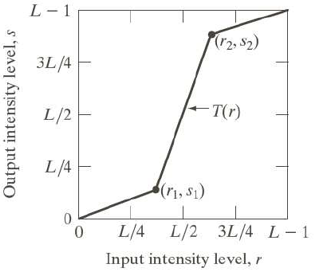### Bit-plane Slicing

As discussed previously, each pixel of a grayscale image is stored as a 8-bit byte. The intensity spans from 0 to 255, which is ‘00000000’ to ‘11111111’ in binary.

Bit-plane slicing refers to the process of slicing a matrix of 8 bits into 8 planes.

The most left bit (the 8th bit from right to left) carries the most weight so it is called the Most Signification Bit. The most right bit (the 1st bit from right to left) carries the least weight so it is called the Least Signification Bit.

Histogram equalization, also known as grayscale equalization, is a practical histogram correction technique.

It refers to the transformation where an output image has approximately the same number of pixels at each gray level, i.e., the histogram of the output is uniformly distributed. In the equalized image, the pixels will occupy as many gray levels as possible and be evenly distributed. Therefore, such image has a higher contrast ratio and a larger dynamic range.

This method is to boost the global contrast of an image to make it look more visible.

The general histogram equalization formula is: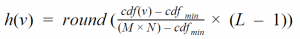• CDF refers to the cumulative distribution function
• L is the maximum intensity value (typically 256)
• M is the image width and N is the image height
• h (v) is the equalized value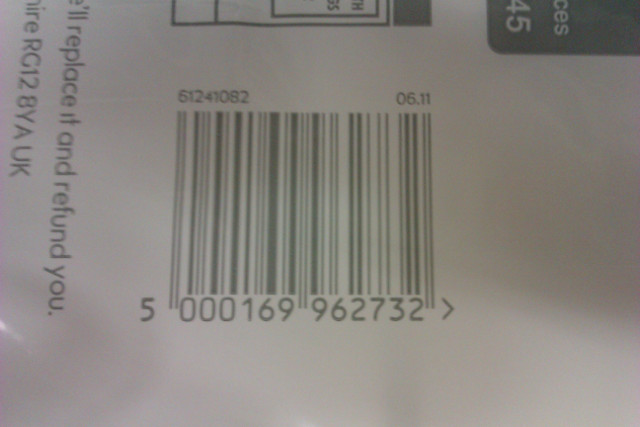Before Histogram Equalization 🠕Histogram Chart Before Equalization 🠕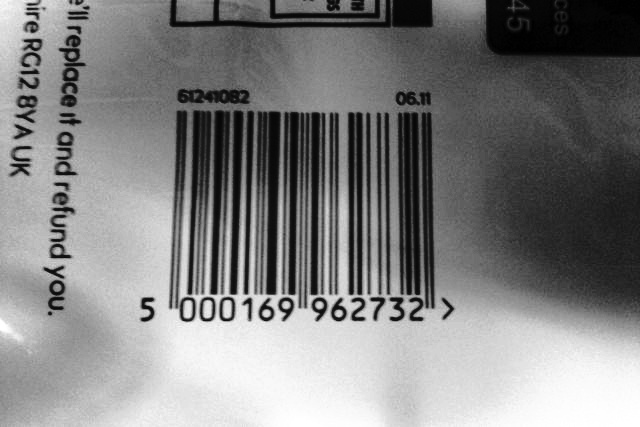After Histogram Equalization 🠕Histogram Chart After Equalization 🠕